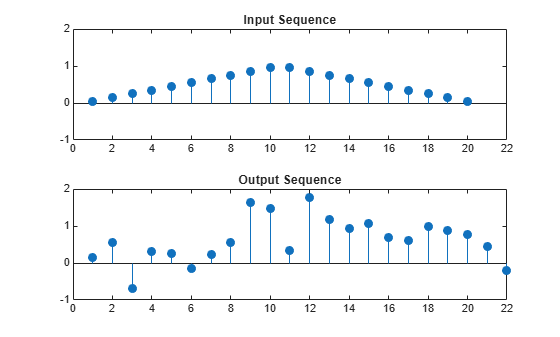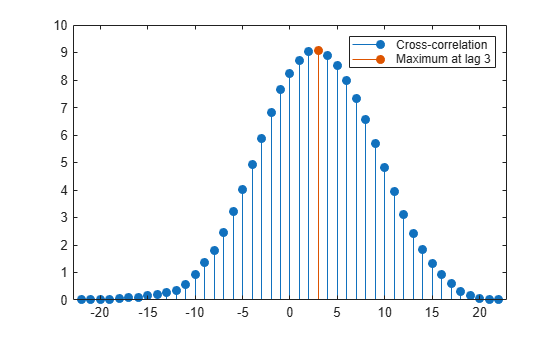Documentation

Cross-Correlation of Delayed Signal in Noise

This example shows how to use the cross-correlation sequence to detect the time delay in a noise-corrupted sequence. The output sequence is a delayed version of the input sequence with additive white Gaussian noise. Create two sequences. One sequence is a delayed version of the other. The delay is 3 samples. Add $N\left(0,0.{3}^{2}\right)$ white noise to the delayed signal. Use the sample cross-correlation sequence to detect the lag.

Create and plot the signals. Set the random number generator to the default settings for reproducible results.

rng default

x = triang(20);
y = [zeros(3,1);x]+0.3*randn(length(x)+3,1);

subplot(2,1,1)
stem(x,'filled')
axis([0 22 -1 2])
title('Input Sequence')

subplot(2,1,2)
stem(y,'filled')
axis([0 22 -1 2])
title('Output Sequence')Obtain the sample cross-correlation sequence and use the maximum absolute value to estimate the lag. Plot the sample cross-correlation sequence.

[xc,lags] = xcorr(y,x);
[~,I] = max(abs(xc));

figure
stem(lags,xc,'filled')
legend(sprintf('Maximum at lag %d',lags(I)))
title('Sample Cross-Correlation Sequence')The maximum cross correlation sequence value occurs at lag 3 as expected.